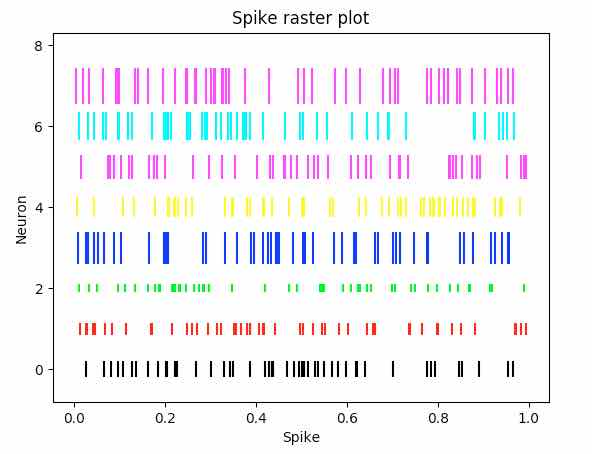# Plotting Neural Events With Spike Raster Plot Using Python Matplotlib

## Overview:

• A Spike raster plot is a plot used in neuroscience to study the neural responses.

• Neurons generate spikes or action potentials in response to various stimuli.

• The Spike raster plot marks the neural activity - either a spike or an action potential from a neuron at a specified position.

• The Y-axis of the spike raster plot can represent either a neuron or a trial number of the experiment on a specific neuron.

• The X-axis of the spike raster plot represents the spike.

• The eventplot() function matplotlib.pyplot module marks lines at specified locations.

• If the orientation of the plot is horizontal the lines drawn are vertical lines.

• If the orientation of the plot is vertical the lines drawn are horizontal lines.

• The line drawn extends to both sides of the Y-axis or the X-axis (when orientation of the plot is vertical) with equal height or width.

• The size of the line is controlled by the linelengths parameter.

## Example:

 import matplotlib.pyplot as plot import numpy as np   # Set the random seed for data generation np.random.seed(2)   # Create rows of random data with 50 data points simulating rows of spike trains neuralData = np.random.random([8, 50])   # Set different colors for each neuron colorCodes = np.array([[0, 0, 0],                         [1, 0, 0],                         [0, 1, 0],                         [0, 0, 1],                         [1, 1, 0],                         [1, 0, 1],                         [0, 1, 1],                         [1, 0, 1]])                         # Set spike colors for each neuron lineSize = [0.4, 0.3, 0.2, 0.8, 0.5, 0.6, 0.7, 0.9]                                            # Draw a spike raster plot plot.eventplot(neuralData, color=colorCodes, linelengths = lineSize)        # Provide the title for the spike raster plot plot.title('Spike raster plot')   # Give x axis label for the spike raster plot plot.xlabel('Neuron')   # Give y axis label for the spike raster plot plot.ylabel('Spike')   # Display the spike raster plot plot.show()

## Output: